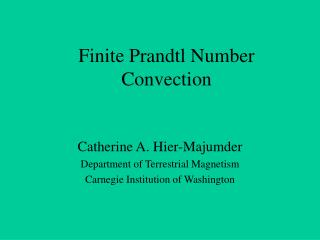DownloadDownload PresentationFinite Prandtl Number Convection

# Finite Prandtl Number Convection

Télécharger la présentation## Finite Prandtl Number Convection

- - - - - - - - - - - - - - - - - - - - - - - - - - - E N D - - - - - - - - - - - - - - - - - - - - - - - - - - -
##### Presentation Transcript

1. Finite Prandtl Number Convection Catherine A. Hier-Majumder Department of Terrestrial Magnetism Carnegie Institution of Washington

2. Collaborators • University of Minnesota, Minneapolis: David A. Yuen • Université de Montréal: Alain P. Vincent

3. Purpose of Study • Many fluids with large, but finite Pr numbers (Pr~104) in geophysical systems (mushy ice, Mg-magmas) • Usually approximated as infinite Pr, same as mantle (Pr~1025) • Pr~104 fluids still have significant inertial behaviors missed by infinite Pr approximation

4. Outline • Equations of Finite Prandtl Convection • 2-D Finite Prandtl Plumes • Infinite vs. finite Prandtl plumes • 3-D Finite Prandtl Plumes • Conclusions

5. Finite Prandtl Convection Equations Nondimensionalized by k

6. Finite Prandtl Convection Equations

7. Nondimensional Temperature Red is hot T > 1 Blue is cold T ~ 0 Ra = 107, Pr = 7 Ra = 107, Pr = 7

8. Nondimensional Temperature Red is hot T > 1 Blue is cold T ~ 0 Ra = 107, Pr = 7

9. Nondimensional Temperature Red is hot T > 1 Blue is cold T ~ 0 Ra = 108, Pr = 2 x 104

10. Nondimensional Temperature Red is hot T > 1 Blue is cold T ~ 0 Ra = 108, Pr = ∞

11. Ra = 108, Pr = infinity t = 2t1 Ra = 108, Pr = 2 x 104 t = t1

12. Implications for Plume Heat Flux • Heat flux = vz * T • Real heat fluxes are likely to be at least 2X larger than those calculated using infinite Pr approximation

13. Conclusions • Prandtl number 104 plumes have significantly different behaviors from infinite Prandtl number plumes: • Hotter • Grow faster • Differences increase with Rayleigh number • This may have significant effects on heat fluxes in icy satellites, very hot bodies like Io, and magma ocean phases of the early terrestrial planets and moons

14. Ra = 6.57 x 105, Pr = 10

15. Questions?